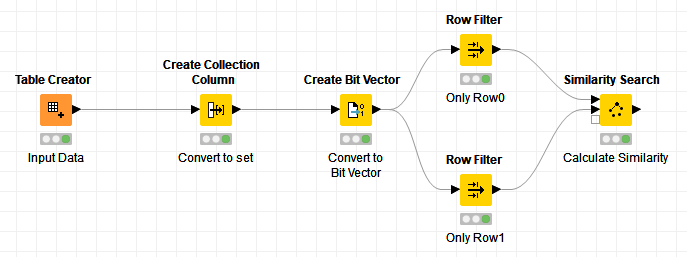# Jaccard similarity coefficient

Hello
How one can calc Jaccard similarity coefficient between rows that contains “strings” ?
Best
Malik

Hi @malik -

Here’s an example workflow that uses a toy dataset. It compares a series of strings by first converting the strings to a collection, then converting that collection to a bitvector, and using the Similarity Search node to calculate (1 - Tanimoto distance), which is effectively the Jaccard similarity coefficient.

In this case you can verify by hand that we have 1 string intersection among 9 values in the union, so the coefficient is 1/9 = 0.111.JaccardTanimotoExample.knwf (14.1 KB)

2 Likes